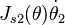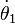Modern Robotics Errata

(Difference between revisions)

Errata below are for the preprint version posted on May 3, 2017. Thanks to everyone who provided corrections to the previous version!

Significant corrections

Chapter 5

• Equation (5.7): The second term on the right-hand side should be$J_{s2}(\theta) \dot{\theta}_2$.

Chapter 6

• Chapter 6.3, first line after Eq (6.7): the matrices$T_{sd}^{-1} \dot{T}_{sd}$ and$\dot{T}_{sd} T_{sd}^{-1}$ are referred to as twists, but these are the se(3) matrix representations of the twists.

Chapter 8

• Figure 8.5 says the volume of the rectangular parallelepiped is abc but it should be hlw.

Minor typos, etc., no danger of misunderstanding

Chapter 1

• (online version only) Description of Chapter 6: "jont positions" should be "joint positions."

Chapter 2

• Chapter 2.2.2, Example 2.3: "Substituting" is misspelled.

Chapter 6

• (online version only) Figure 6.7: θ0 should be θ0 (five times) and θ1 should be θ1 (one time).

Chapter 7

• Just below caption for Figure 7.8: There is an extraneous dot.

Chapter 8

• (online version only) Chapter 8.1.1, last paragraph:$\dot{\theta}_1$ is improperly typeset.